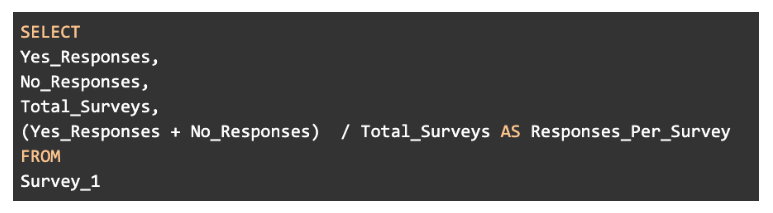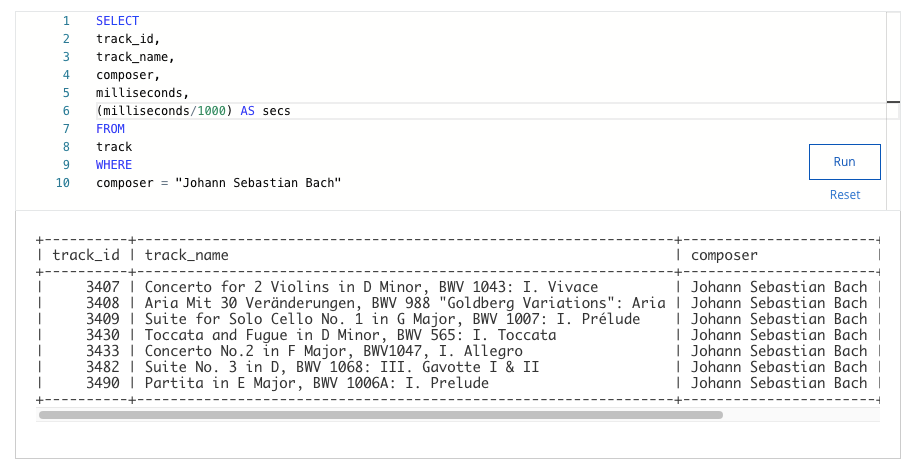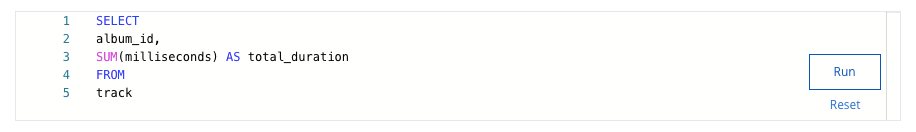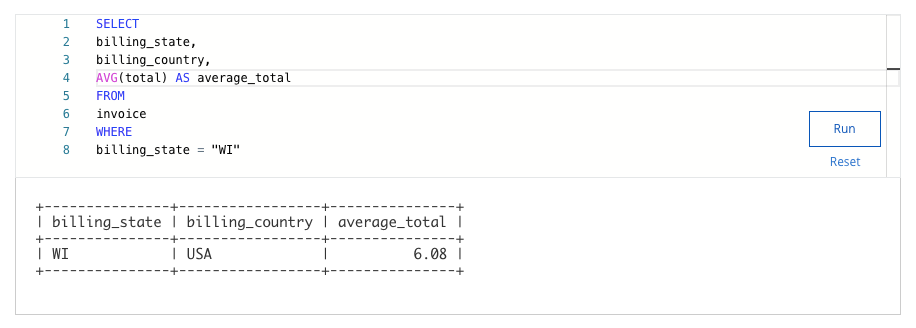# analyze data to answer questions weekly challenge 4

#### 1. A data analyst is working with a spreadsheet from a furniture company. To use the template for this spreadsheet, click the link below and select “Use Template.” Link to template: Sample Transaction Table.Or, if you don’t have a Google account, download the file directly from the attachment below.The syntax of which of the following formulas would allow the analyst to count purchase sizes of two or more?

• =COUNTIF(G2:G30, “>=2”)
• =COUNTIF(H2:H30, “>=2”)
• =SUMIF(H2:H30, “=4”)
• =SUMIF(G2:G30, “<=1”)

• ”<10”
• =SUMIF
• C1:C25
• A1:A25

#### 3. A data analyst is working in a spreadsheet and uses the SUMPRODUCT function in the formula below as part of their analysis. =SUMPRODUCT(A2:A10,B2:B10)How does the SUMPRODUCT function calculate the cell ranges identified in the parentheses?The analyst wants to figure out the value of all of the items in the spreadsheet. Which formula will calculate the total price of all of the items?

• It multiplies the values in the first range, then multiplies the values in the second range .
• It adds the values in the first range, then adds the values in the second range.
• It multiplies the ranges, then adds the sum of the products of the two ranges.
• It adds the ranges, then multiplies them by the last value in the second array.

#### 4. You create a pivot table in a spreadsheet containing movie data. To use the template for this spreadsheet, click the link below and select “Use Template.” Link to template: Movie Data Project.Or, if you don’t have a Google account, download the file directly from the attachment below.If you want to summarize the data using the AVERAGE function in the Values menu, which spreadsheet columns could you add data from? Select all that apply.

• Box Office Revenue
• Movie Title
• Genre
• Budget

#### 5. A data analyst uses the following SQL query to perform basic calculations on their data. Which types of operators is the analyst using in this SQL query? Select all that apply.• Multiplication
• Subtraction
• Division

#### 6. You are working with a database table that contains data about music. The table includes columns for track_id, track_name, composer, and milliseconds (duration of the music track). You are only interested in data about the classical musician Johann Sebastian Bach. You want to know the duration of each Bach track in seconds. You decide to divide milliseconds by 1000 to get the duration in seconds, and use the AS command to store the result in a new column called secs. Add a statement to your SQL query that calculates the duration in seconds for each track and stores it in a new column as secs.NOTE: The three dots (...) indicate where to add the statement.• 307
• 120
• 153
• 193

#### 7. You are working with a database table that contains data about music. The table includes columns for album_id and milliseconds (duration of the music tracks on each album). You want to find out the total duration for each album in milliseconds, and store the result in a new column named total_duration. You write the SQL query below. Add a GROUP BY clause that will group the data by album Id number.• 257252
• 959711
• 342562
• 858088

#### 8. You are working with a database table that contains invoice data. The table includes columns for billing_state, billing_country, and total. You want to know the average total price for the invoices billed to the state of Wisconsin. You decide to use the AVG function to find the average total, and use the AS command to store the result in a new column called average_total. Add a statement to your SQL query that calculates the average total and stores it in a new column as average_total.NOTE: The three dots (...) indicate where to add the statement.• 5.54
• 5.78
• 6.08
• 5.37

## Shuffle Q/A 1

#### 9. A data analyst wants to calculate the number of rows that have a value of “shipped”. Which function could they use?

• =MAX(G2:G30,”=shipped”)
• =SUM(G2:G30,”=shipped”)
• =COUNT(G2:G30,”=shipped”)
• =COUNTIF(G2:G30,”=shipped”)

• E2:E10
• >=50
• D2:D10
• =SUMIF